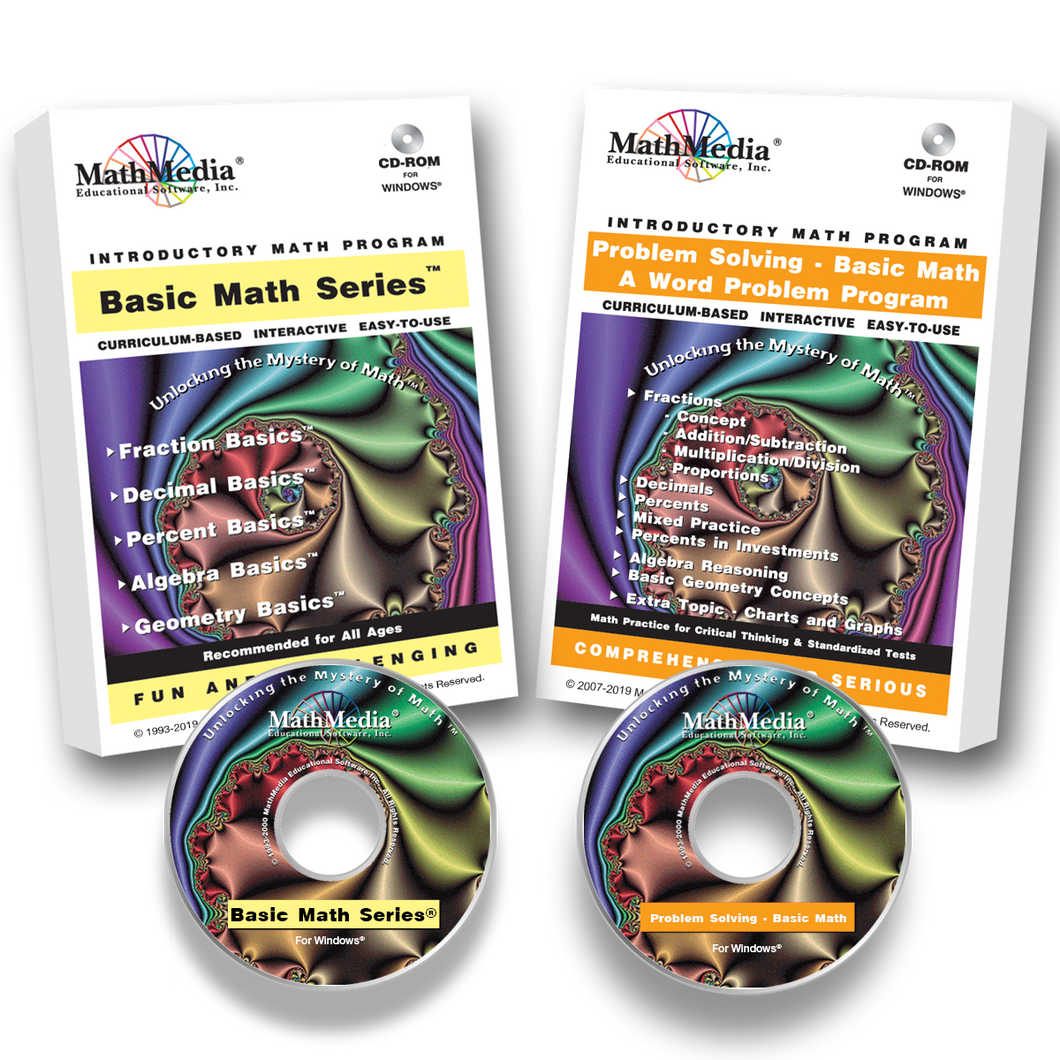# Basic Math Series PLUS Word Problems

Regular price \$189.00 \$47.25 Sale

Purchase the 5-program Basic Math Series PLUS the Word Problems for Basic Math.

The Basic Math Series includes 5 programs:

FRACTION BASICS®
DECIMAL BASICS®
PERCENT BASICS®
ALGEBRA BASICS®
GEOMETRY BASICS®

The basic math course covers the Standards for fractions, decimals, percents, and an introduction to Algebra and Geometry. This is the math curriculum for Grade 4 through adult education courses. Use the MathMedia Basic Math software system to learn, re-learn, review, and prepare for proficiency exams and achievement tests. Basic math skills are introduced, practiced, and mastered in an easy-to-use, menu-driven system of instruction, practice, and assessments.

Basic Math Series Curriculum SyllabusEach basic math skills program offers interactive math problems with solutions and explanations. Help is always a click away. Hint screens unfold step by step to allow the student to see the problem solving process.

In addition to mini-quizzes for each section, concluding comprehensive tests for all five (5) basic math software programs are included. These tests are scored and stored by the computer for evaluation of progress -- A valuable tool for the middle school math teacher as well as those facilitating the learning of adult math students. After taking the test, a personalized score sheet is printed along with an evaluation of topics requiring further study. The student can then return to the body of the program and practice more math problems in those sections which were weak areas. The use of the tests is flexible -- the student may take Test A as a pre-test and Test B as a post-test or Test A may be used for one student and Test B for another.

Learning Management System (LMS)
The LMS automatically tracks students' test scores and provides the teacher with a database (.csv file) to sort and print as needed. Student competencies and weaknesses are recorded for analysis and to direct the student to those sections of the program which require more practice.

These programs automatically bookmark the student's last viewed screen and will prompt the student to return to that screen upon their return.

Word Problems for Basic Math:

This program concentrates ONLY on word problems to test the skills learned in the first five programs and prepare for standardized tests. Give students the opportunity to prepare thoroughly and comprehensively for not only standardized math tests but also to be a strong math student. "The more you know, the farther you go!"
This is an interactive basic math problem solving program for math students who have mastered the mechanics of the operations on fractions, decimals, percents, pre-algebra, and intro-geometry. With over 200 menu-driven practice problems, students will be able to focus on reading the words, deciphering the meaning, and applying what they know about basic math problem solving. Students will learn math problem-solving strategies and practice the types of math word problems, which typically appear on standardized tests. Hints are available to help and coach the student along. Score reports are generated at the end of each section.
This math problem solving program is divided into topic sections, so that a student who has mastered fractions but not yet pre-algebra can still work on the fraction section before tackling the pre-algebra section. These math word problems are geared toward teaching and learning problem solving techniques on the types of questions encountered on standardized tests given to 5th grade math through 8th grade math students as well as typical everyday life math problems.
Students must practice and master the appropriate basic math skills program before or concurrently with the problem solving program.